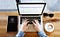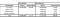pixabay

# The Best Way to Do Cap Table Math (In My Opinion…)

Cap table math is confusing. Determining the post-money cap table for an equity round with an option pool refresh and one or more convertible notes converting to equity can be overwhelming. Over the years, I’ve encountered numerous shortcuts to tame the complexity. While they simplify the calculations, they often yield incorrect results because the logic isn’t obvious and it’s easy to make faulty assumptions.

[Note: There are two commonly used methods for converting convertible notes. The first method assumes notes convert simultaneously with the equity investment, so that the equity investors and note investors dilute each other. The second method assumes that notes convert prior to the equity investment, so that the note investors do not dilute the equity investors. This is more dilutive for founders. This post focuses on the latter method, which requires more complicated math and is more error-prone in my experience. This post from Capshare does a nice job of describing the methods and my eBook The Founder’s Guide to Venture Capital Finance provides significant detail and example both methods]

I’ll begin by showing why shortcuts can be problematic and then develop my recommended methodology for cap table math.

It’s Easy to Get Confused

I want to illustrate how calculation shortcuts can lead to unintended assumptions. One of the most ubiquitous shortcuts is one I’ll call the “effective pre-money method”. To use this method, determine a dollar value for the option pool and convertible notes and subtract them from the pre-money valuation. This yields an “effective pre-money valuation”. Divide the effective pre-money valuation by the pre-money shares and, voila: share price. Everything else falls in line. Let me illustrate with an example:

Example

ABC Co. raises a \$2M financing at an \$8M pre-money valuation. The pre-money valuation assumes a 15% option pool refresh and conversion of all convertible notes.

• Currently the Founders own 100% of the shares. There are 10M shares outstanding.
• The company has \$500K in convertible debt (principal + interest) with a 20% discount and \$4M cap.

The effective pre-money methodology applies the following logic:

Effective Pre-Money Valuation = Stated Pre-Money Valuation — Value of Option Pool — Value of Notes

Value of Option Pool = Option Pool % x Post-Money Valuation = 0.15 x \$10M = \$1.5M

What about the value of the notes? I’ve seen this handled a variety of ways. Which of the following is correct?

A) Value of Notes = (\$500K / (\$4M + \$500K)) = \$1.11M
B)
Value of Notes = (\$500K / (\$4M + \$500K)) x \$8M = \$0.88M
C)
Value of Notes = (\$500K / \$4M) x \$8M = \$1.00M
D)
Value of Notes = (\$500K / \$4M) x \$10M = \$1.25M

It was a trick question. None of those options yields what I consider to be the correct answer. All of the formulas above neglect the fact that the option pool dilutes the noteholders. Two of them — Options C and D — assume a post-money valuation cap which, at the time of writing, is highly uncommon. The math is simple, but the answer is wrong. To account for the impact of the option pool:

Value of Notes = (Principal + Interest) / (Principal + Interest + Cap) x (Pre-Money Valuation — Value of Option Pool)

Value of Notes = (\$500K / (\$500K + \$4M)) x (\$8M — \$1.5M) = \$0.72M

This was a long digression, but I wanted to illustrate how easy it can be to miscalculate using simplified methods. Now, I’m going to introduce my recommended method for cap table calculations. You’ll have to do some algebra, but I believe the underlying logic is much clearer than that of any of the “shortcut methods”.

My Recommended Method

I’ve done dozens (probably hundreds) of these calculations over the years. In my experience, the best method — and least subject to misinterpretation — is to frame the problem in terms of shares rather than dollars. Begin by calculating post-money shares and determine all of the other values with a few simple formulas.

Assuming the company raises an equity round with notes converting and an option pool refresh:

Post-Money Shares = Pre-Money Shares + Equity Investor Shares + Noteholder Shares + New Options

I hope the logic is clear. The total shares after the financing round must equal the shares prior to the financing round plus any new shares. Now, develop definitions for each term in the formula above.

Pre-Money Shares
Pre-money shares is the total shares prior to the financing round. It includes Founders’ shares and may include an existing option pool or shares issued to previous investors.

Equity Investor Shares
Equity investor shares represents the shares issued to new direct equity investors.

Equity Investor Shares = Equity Investment / Share Price

Since:

Share Price = Post-Money Valuation / Post-Money Shares

We can restate Equity Investor Shares as:

Equity Investor Shares = (Equity Investment x Post-Money Shares) / Post-Money Valuation

Noteholder Shares
Noteholder shares are the shares issued to noteholders when their convertible debt converts to equity.

If the notes convert at a discount to the equity financing

Noteholder Shares = (Principal + Interest) / (Share Price x (1 — Discount %))

Using the same definition for Share Price stated above:

Noteholder Shares = (Principal + Interest) x Post-Money Shares / (Post-Money Valuation x (1 — Discount %)

If the notes convert at a valuation cap

Noteholder Shares = (Principal + Interest) / (Valuation Cap / Pre-Money Shares)

New Options
Investors typically require an unallocated option pool equal to some percentage of the post-money shares. For instance, investors may require a 15% option pool. If the company already has an existing option pool, the number of new shares added to the pool will be less than 15% of the post-money shares. New options represents any additional options reserved for the option pool refresh.

New Options = Option Pool % x Post-Money Shares — Available Options

Where Available Options represents the unallocated options in the existing pool and Option Pool % is the target post-money option pool percentage.

Tying Everything Together
Now that we’ve developed each of the components, we can pull it together. For the sake of specificity, assume the notes convert at a discount in this scenario.

Post-Money Shares = Pre-Money Shares + Equity Investor Shares + Noteholder Shares + New Options

Post-Money Shares = Pre-Money Shares + (Equity Investment x Post-Money Shares) / Post-Money Valuation + (Principal + Interest) x Post-Money Shares / (Post-Money Valuation x (1 — Discount %)) + Option Pool % x Post-Money Shares — Available Options

Okay, so that’s a really ugly equation. However, notice that every term on the right side of the equation is multiplied by Post-Money Shares:

Post-Money Shares = Pre-Money Shares + (Equity Investment x Post-Money Shares) / Post-Money Valuation + (Principal + Interest) x Post-Money Shares / (Post-Money Valuation x (1 — Discount %)+Option Pool % x Post-Money Shares — Available Options

Subtract all of those terms from both sides and factor out Post-Money Shares:

Post-Money Shares x (1 — Equity % — Noteholder % — Option Pool %) = (Pre-Money Shares — Available Options)

Where:

Equity % = Equity Investment / Post-Money Valuation

Noteholder % = (Principal + Interest) / (Post-Money Valuation x (1 — Discount %))

Then solve for Post-Money Shares:

Post-Money Shares = (Pre-Money Shares — Available Options) / (1 — Equity % — Noteholder % — Option Pool %)

Knowing the total post-money shares, it’s simple to develop the post-money cap table using the definitions for equity investor shares, noteholder shares, etc., derived above. Here is a simple example to make this more concrete:

Example

ABC Co. raises a \$2M financing at an \$8M pre-money valuation. The pre-money valuation assumes a 15% option pool refresh and conversion of all convertible notes.

• Currently the Founders own 100% of the shares. There are 10M shares outstanding.
• The company has \$500K in convertible debt with a 20% discount and \$4M cap.

Pre-Money Shares = 10M

Equity % = Equity Investment / Post-Money Valuation = \$2M / \$10M = 0.20

Noteholder Shares = (Principal + Interest) / (Valuation Cap / Pre-Money Shares) = \$500K / (\$4M / 10M) = 1.25M

Option Pool % = 0.15

Available Options = 0

Post-Money Shares = (Pre-Money Shares + Noteholder Shares) / (1- Equity % — Option Pool %)

Post-Money Shares = (10M + 1.25M) / (1–0.20–0.15) = 17,307,692

Then:

Equity Investor Shares = Equity Investment / (Post-Money Valuation / Post-Money Shares)

Equity Investor Shares = \$2M / (\$10M / 17,307,692) = 3,461,538

Option Pool = Option Pool % x Post-Money Shares = 0.15 x 17,307,692
Option Pool = 2,596,154

The pre-money and post-money cap tables appear below. The Google Sheet here shows the calculations. You can download it and play around with different inputs (shaded in yellow) to see how they affect the outcome:Pre-money and Post-money cap tables

Conclusion

My recommended method requires more algebra than some of the shortcut methods, but by making the logic explicit you’re more likely to obtain a reasonable result. Furthermore, it’s easy to extend or condense this method to fit different scenarios. No option pool refresh? Eliminate that term from the formula. No convertible notes? Eliminate that term too. Multiple convertible notes with different caps or discounts? Add each of them independently:

Post-Money Shares = Pre-Money Shares + Equity Investor Shares + Noteholder #1 Shares + Noteholder #2 Shares + Noteholder #3 Shares + …..+ Noteholder #N Shares + New Options

In every case:

• Begin by defining post-money shares.
• Next, define each of the individual components in terms of post-money shares.
• Finally, subtract from both sides of the equation, factor our post-money shares, and solve.
• After determining post-money shares, it’s simple to calculate share price and determine shares issued to equity investors, noteholders, etc., based on the definitions above.

This is probably one of the more boring, tactical posts I’ll ever write, but I think this is important information for Founders to understand. I hope this is helpful.

Note: I published an ebook called “The Founder’s Guide to Venture Capital Finance” that cover this topic, and all of the other calculations you should understand when raising money from VCs and angel investors. It includes more detail and lots of step-by-step examples. Check it out.

Written by

## Startups and investment

#### Posts from experts at Innovation Works.

Welcome to a place where words matter. On Medium, smart voices and original ideas take center stage - with no ads in sight. Watch
Follow all the topics you care about, and we’ll deliver the best stories for you to your homepage and inbox. Explore
Get unlimited access to the best stories on Medium — and support writers while you’re at it. Just \$5/month. Upgrade